# Kontorovich-Lebedev transform

Lebedev–Kontorovich transformwhereis the Macdonald function.

This transform was introduced in [a1] and later investigated in [a2]. Ifis an integrable function with the weight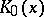, i.e.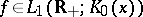, then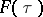is a bounded continuous function, which tends to zero at infinity (an analogue of the Riemann–Lebesgue lemma, cf. Fourier series, for the Fourier integral). Ifis a function of bounded variation in a neighbourhood of a point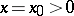and if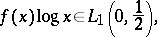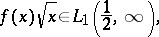then the following inversion formula holds:If the Mellin transform of, denoted by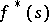, belongs to the space, thencan be represented by an integral (see [a6]):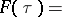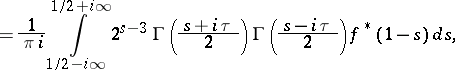where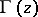is the Euler gamma-function.

Let. Then the integralconverges in mean square and isomorphically maps the space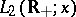onto the space. The inverse operator has the form [a10]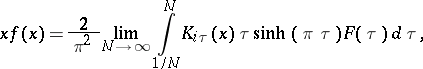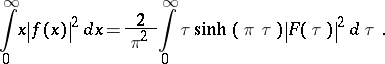The Kontorovich–Lebedev transform of distributions was considered in [a7], [a8]. A transform table for the Kontorovich–Lebedev transform can be found in [a4]. Special properties in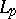-spaces are given in [a10].

For two functions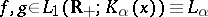,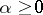, define the operator of convolution for the Kontorovich–Lebedev transform as ([a9], [a10])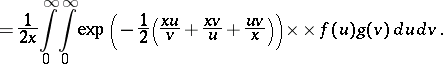The following norm estimate is true: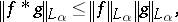and the space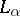forms a normed ring with the convolution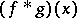as operation of multiplication.

If,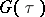are the Kontorovich–Lebedev transforms of two functions,, then the factorization property is true: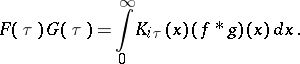If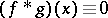in the ring, then at least one of the functions,is equal to zero almost-everywhere on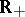(an analogue of the Titchmarsh theorem).

The Kontorovich–Lebedev transform is the simplest and most basic in the class of integral transforms of non-convolution type, which forms a special class of so-called index transforms (cf. also Index transform), depending upon parameters, subscripts (indices) of the hypergeometric functions (cf. Hypergeometric function) as kernels.